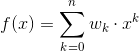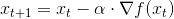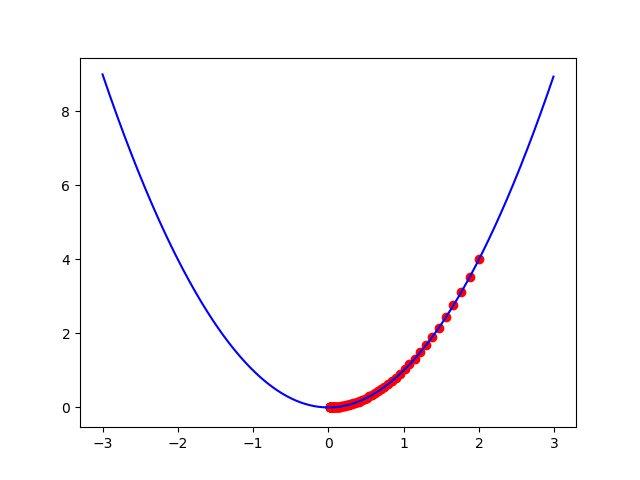#2019 iT 邦幫忙鐵人賽DAY 28
0
AI & Data

## Tabular Method

• 說起來很簡單，但做起來就不一定了。
• 其實之前在陳述狀態價值時，有時會筆誤成狀態價值函數，原因是因為大多的方法已經不用表格記錄，而是使用函數逼近，這種情況下的狀態價值會由 狀態價值函數 估計。(這絕對不是在幫自己的筆誤找藉口)

## Function Approximation (函數逼近)

• Coarse Coding
• Tile Coding
• Kanerva Coding• t 表示第幾次迭代
•一樣是學習率 (從誤差更新多少)
• 實作如下
``````import numpy as np
import matplotlib.pyplot as plt

# function
def func(x):
return np.power(x,2)

def dfunc(x):
return 2*x

def GD(x_start, alpha, threshold):
trajectory = [x_start]
x = x_start
update = float('Inf')
while abs(update) > threshold:
dx = dfunc(x)
update = -dx*alpha
x += update
trajectory.append(x)
return trajectory

# main
x = GD(2, 0.03, 0.001)

# plot
t = np.arange(-3, 3, 0.01)
plt.plot(t, func(t), color = 'b')
plt.scatter(x, func(x), color = 'r')
plt.show()
``````
• 結果圖